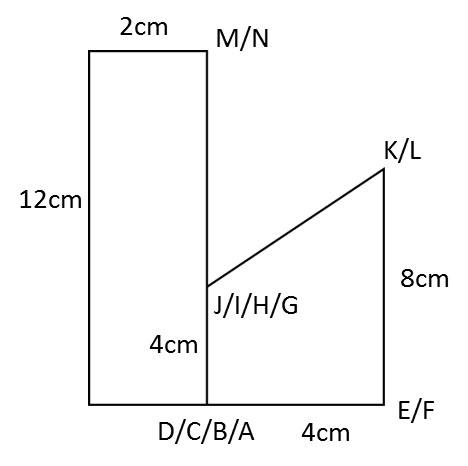# 10.3.2 Plan and Elevation, SPM Paper 2 (Long Questions)

Question 3:The diagram above shows a right prism attach to a cuboid at one of its plane. ABCG and CDEF, IJNH and KLMJ are horizontal planes, ABIH, AGNH and GCJN are vertical planes while IBCJ is an inclined plane. HA = 3 cm, BC = 3 cm, CD = 1.5 cm, HI = NJ = JK = 3 cm. Draw to full scale

(a) The elevation of the solid on a vertical plane parallel to AB as viewed from X.(b) A solid semi-cylinder is joined to the solid in A as show in the diagram above. JPON is a vertical plane and JP = 1.5 cm. Draw to full scale
i. The plan of the solid
ii.   The elevation of the solid on a vertical plane parallel to BCD as viewed from Y.

Solution:

(a)(b)(i)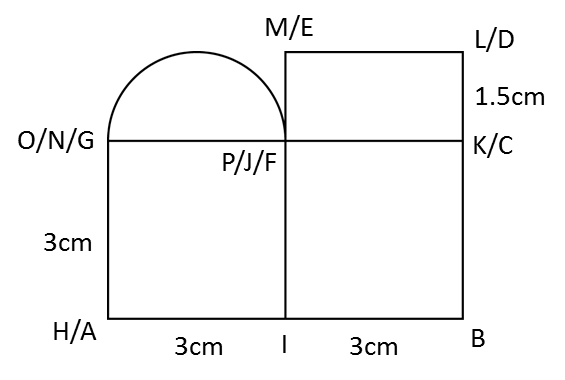(b)(ii)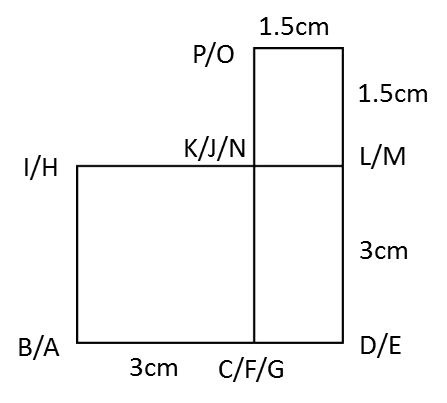Question 4: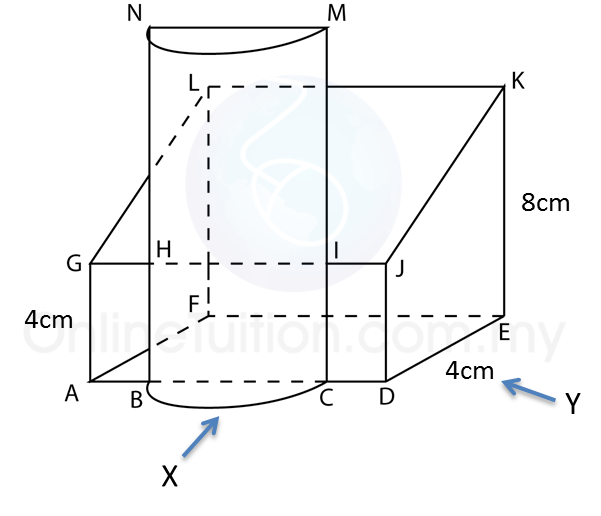The diagram above shows a solid consisting of a right prism and a half-cylinder which are joined at the plane HICB. The base ABCDEF is on a horizontal plane. The rectangle LKJG is an inclined plane. The vertical plane JDEK is the uniform cross-section of the prism. AB = CD = 2 cm. BC = 4 cm. CM = 12 cm.
Draw to full scale
(a)  The plan of the solid
(b) The elevation of the solid on a vertical plane parallel to ABCD as viewed from X.
(c)  The elevation of the solid on a vertical plane parallel to DE as viewed from Y.

Solution:
(a)(b)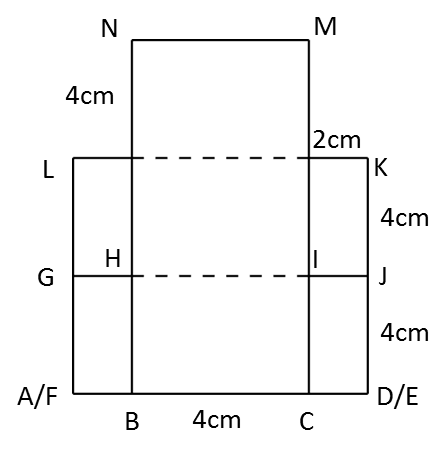(c)